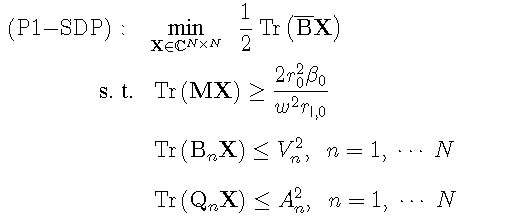# CVX/sedumi solves a SDP prblem,but the result is unreasonable and one constraint is not satisfied

Hello,everyone.
Recently, I am trying to write my own matlab /cvx code on a SDP promblem based on a published paper. I have used the sedumi solver, and set the precision high. At first glance, It seems that I have solved the proplem successfully since the status is ’ solved ‘. However, compared with the result derived by the author , I’ve got far less values, which come to 10^-8 , while the normal values should be among 10 to 100, approximately. What’s worse, the solution doesn’t satisfy the first inequality constraint! I’m sure that numerical calculations and variable initializations are correct.
I will show you the SDP problem and my code.The key code in matlab main function:
`indent preformatted text by 4 spaces`
n = 5;
omiga = 6.78 * 2* pi * 10^6;
rr = 50.336;
rt = [0.336, 0.336, 0.3336, 0.336, 0.336];
m = [1.6121, 0.00781, -0.0296, 0.00781, 0.1508].’* 10^-6;
M = [5886.8, 0.3565, 0.1253, 0.3565, 0.2984;
0.3565, 5886.8, 0.3565, 0.1253, 0.2984;
0.1253, 0.3565, 5886.8, 0.3565, 0.2984;
0.3565, 0.1253, 0.3565, 5886.8, 0.2984;
0.2984, 0.2984, 0.2984,0.2984, 5886.8] * 10^-6;
powerindex = 5:5:100;
voltage = [1800,1800,1800,1800,1800].’.^(1/2);
current = [50,50,50,50,50].’.^(1/2);
for power_index = 1:length(powerindex)
power = powerindex(power_index);
fprintf(‘circle is running ‘);
fprintf(’%d\n’,power_index);
[X] = METHOD_SDP_COPY(rt, rr, rl, m, power, current, voltage, omiga, n, M);
end
`indent preformatted text by 4 spaces`
function METHOD_SDP_COPY:
`indent preformatted text by 4 spaces`
function [X] = METHOD_SDP_COPY(rt, rr, rl, m, power, current, voltage, omiga, n, M)
M_ = zeros(n,n);
M_= m * m’;
B = zeros(n, n);
B_ = zeros(n, n);
B__ = zeros(n, n);
BN = zeros(n, n, n);
QN = zeros(n, n, n);
for p = 1 : n
for q = 1 : n
if p == q
B_(p, q) = rt§ + m§^2 * omiga^2 / rr;
B__(p, q) = 0;
B(p, q) = B_(p, q) + 1i * B__(p, q);
else
B_(p, q) = m§ * m(q) * omiga^2 / rr;
B__(p, q) = -omiga * M(p, q);
B(p, q) = B_(p, q) + 1i * B__(p, q);
end
end
end
for i = 1 : n
BN(:, :, i) = B(: , i) * B(:, i)’;
end
for i = 1 : n
QN(i, i, i) = 1;
end
cvx_solver sedumi
cvx_begin sdp
cvx_precision high
variable X(n, n) hermitian
minimize real((1 / 2 * trace(B_* X)));
subject to
real(trace(M_ * X)) >= 2 * rr^2 * power / (omiga^2 * rl);
for i = 1 : n
real(trace( BN(:, :, i) * X )) <= voltage(i)^2;
end
for i = 1 : n
real(trace( QN(: , : , i ) * X )) <= current(i)^2;
end
[ X ] == hermitian_semidefinite( n );
cvx_end
%Determine whether the results out from cvx meet the constraint condition 1
if real(trace(M_ * X)) < 2 * rr^2 * power / (omiga^2 * rl)
fprintf(‘NOTE:After cvx,constr1 is abnormal.\n’);
end
%Determine whether the results out from cvx meet the constraint condition 2
tag1 = 1;
for i = 1 : n
% real(trace( BN(: , : , i ) * X ))
if real(trace( BN(: , : , i ) * X )) > voltage(i)^2
tag1 = 0;
end
end
if tag1 == 0
fprintf(‘NOTE:After cvx,constr2 is abnormal.\n’);
end
%Determine whether the results out from cvx meet the constraint condition 3
tag2 = 1;
for i = 1 : n
real(trace( QN(: , : , i ) * X ))
if real(trace( QN(: , : , i ) * X )) > current(i)^2
tag2 = 0;
end
end
if tag2 == 0
fprintf(‘NOTE:After cvx,constr3 is abnormal.\n’);
end
end

`indent preformatted text by 4 spaces`

A post was merged into an existing topic: CVX status is solved but the result is abnormal and one constraint is not meet